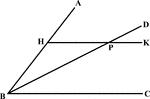### Angle Bisector Drawn Parallel to A Side

Illustration to show that if through any point in the bisector of an angle a line is drawn parallel…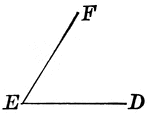### Plane Angle

An illustration of a plane angle with vertex E and sides EF and ED.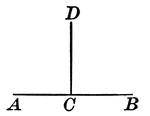### Right Angles With Same Vertex

An illustration showing when one straight line meets another straight line and makes the adjacent angles…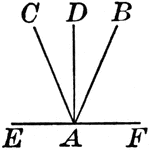### Angles With Same Vertex

An illustration of angles with the same vertex made up of 4 lines.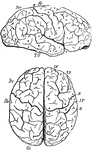### Brain

"Profile and vertex views of cerebrum. Dr, the frontal lobe; Par, parietal; Oc, occipital; Ts, temporo-sphenoidal…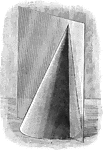### Plane Passing Through the Vertex of a Cone

Illustration of a plane passing through the vertex of a cone (section made is a triangle).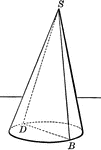### Plane Passing Through the Vertex of a Cone

Illustration of a plane passing through the vertex of a cone (section made is a triangle).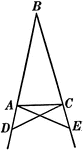### Base Angles In An Isosceles Triangle

Illustration showing that can be used to prove that the base angles of an isosceles triangle are equal.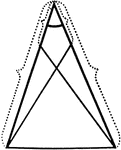### Equal Segments In An Isosceles Triangle

Illustration showing that if equal segments measured from the vertex are laid off on the arms of an…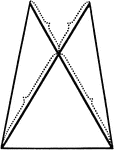### Equal Segments In An Isosceles Triangle

Illustration showing that if equal segments prolonged through the vertex are laid off on the arms of…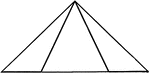### Equal Segments In An Isosceles Triangle

Illustration showing that if equal segments measured from the end of the base are laid off on the base…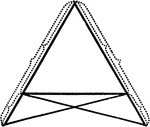### Equal Segments In An Isosceles Triangle

Illustration showing that if equal segments measured from the end of the base prolonged are laid off…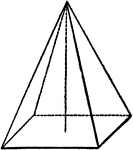### Pryamid

"A pyramid is a solid whose base is a polygon, and whose sides are triangles uniting at a common point,…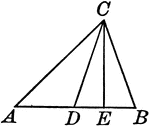### Triangle With Bisector and Perpendicular From Vertex Drawn

Illustration to show the bisector of the vertical angle of a triangle makes with the perpendicular from…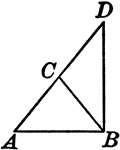### Leg Produced through the Vertex of an Isosceles Triangle

Illustration to show that if one of the legs of an isosceles triangle is produced through the vertex…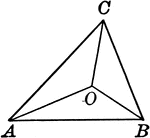### Triangle With Lines From the Vertices That Join a Point Within

Illustration to show lines drawn from the vertices to join at a point within the triangle.